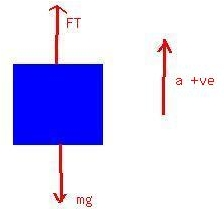# An elevator (mass 4850 kg) is to be designed so that themaximum acceleration is 0.0600g. What is the maximum and the minimum forces the motor should eBrittney Lord 2021-04-26 Answered
An elevator (mass 4850 kg) is to be designed so that themaximum acceleration is 0.0600g. What is the maximum and the minimum forces the motor should exert on the supportingcable?
I already know how to find the maximum force (just multiplyingthe maximum acceleration by the mass), but I've got no clue as tohow to find the minimum acceleration when no minimum accelerationhas been found.
You can still ask an expert for help

• Live experts 24/7
• Questions are typically answered in as fast as 30 minutes
• Personalized clear answers

Solve your problem for the price of one coffee

• Math expert for every subject
• Pay only if we can solve itSadie EatonIn both cases the free body diagram is as shown
Chossing the upward direction as positive,the Maximun tension is obtained by assuming the upward accelerationas positive.
Therefore, $\mathrm{△}F=ma$
${F}_{t}-mg=ma$
Or ${F}_{t}=m\left(a+g\right)$
$=m\left(0.0600g+g\right)$
The minimum acceleration is obtained by assuming theacceleration is down.
Therefore, here ${F}_{t}=m\left(-a+g\right)$
$=m\left(-0.0600g+g\right)$